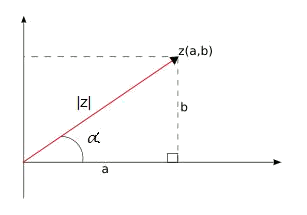# Complex numbers in the polar form: module and argument

Upt ot now we have learnt how to work with complex numbers and we have introduced how to represent them in the complex plane.

What we did was to assign a vector to each complex number, determined by its real and complex part. Thus, what we were essentially doing was to represent vectors on the plane.

However, the vectors can also be represented by means of a longitude and an angle generated with the $$x$$-axis. This is why, the complex number can also be understood as a longitude (the norm of the vector) and angle. Let’s see how it works.

In order to represent a complex number $$z$$ in the polar form we have to use the norm or module and the angle or argument. The module refers to the length of the vector that represents it in the plane, and the argument refers to the angle that it forms with the horizontal axis.

Graphically:Like this we can represent a complex number with its norm and its argument. We will write $$z=|z|_{\alpha}$$ to describe such a number, where:

• $$|z|$$ is the module and it is the square root of the sum of the squares of the real component and the complex component. Usually we write $$|z|$$ or $$r$$ and we can think of it as the distance from the origin to the complex number $$z$$ if we have it represented in the complex plane. Thus, we have: $$|z|=|a+bi|=\sqrt{a^2+b^2}$$$• $$\alpha$$ is called the argument of the complex number $$z$$ and it is the angle formed by the complex number $$z$$ with the real axis (counterclockwise) represented in the complex plane. Thus, we have: $$\alpha=\arctan(\dfrac{b}{a})$$$

We shall notice that the argument of a complex number is not unique, since the expression $$\alpha=\arctan(\frac{b}{a})$$ does not uniquely determine the value of $$\alpha$$, for there are infinite angles that satisfy this identity.

However, if we restrict the value of $$\alpha$$ to $$0\leqslant\alpha< 2\pi$$, there are only two angles that differ in $$\pi$$ and have the same tangent. To know which of them the argument is, we will bear in mind the signs of $$a$$ and $$b$$, thus we will manage to know in what quadrant the vector of the complex number is placed. And it will give us the angle we are looking for.

• If the real part and the complex part are positive, the complex number lives in the first quadrant.

For example $$5+9i$$.

• If the real part is negative and the complex part is positive, the complex number lives in the second quadrant.

For example $$-5+9i$$.

• If the real part and the complex part are negative, the complex number lives in the third quadrant.

For example $$-5-9i$$.

• If the real part is positive and the complex part is negative, the complex number lives in the fourth quadrant.

For example $$5-9i$$.

Let’s find the module and argument of the number $$z=4+4\sqrt{3}i$$.

The complex $$4+4\sqrt{3}i$$ has as module: $$|4+4\sqrt{3}i|=\sqrt{4^2+(4\sqrt{3})^2}=\sqrt{16+16\cdot3}=\sqrt{16+48}= \sqrt{64}=8$$$And the argument is: $$\alpha=\arctan\Big(\dfrac{4\sqrt{3}}{4}\Big) =\arctan(\sqrt{3})=60^{\circ}$$$

Since both the real part and the complex part are positive and therefore the complex number lives in the first quadrant.

Thus, to represent in polar form this complex number, we use: $$z=|z|_{\alpha}=8_{60^{\circ}}$$\$

This methodology allows us to convert a complex number expressed in the binomial form into the polar form.